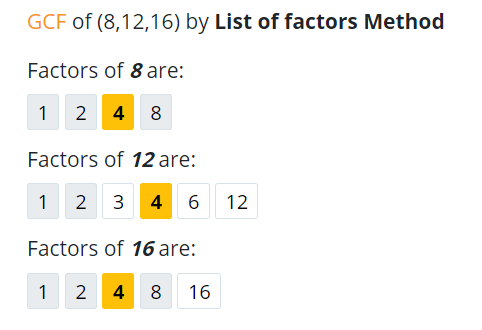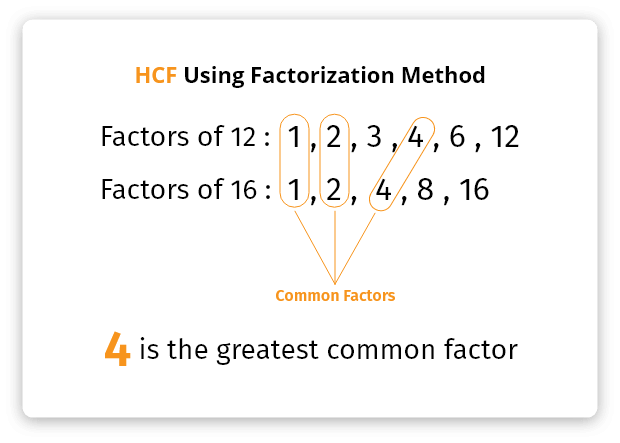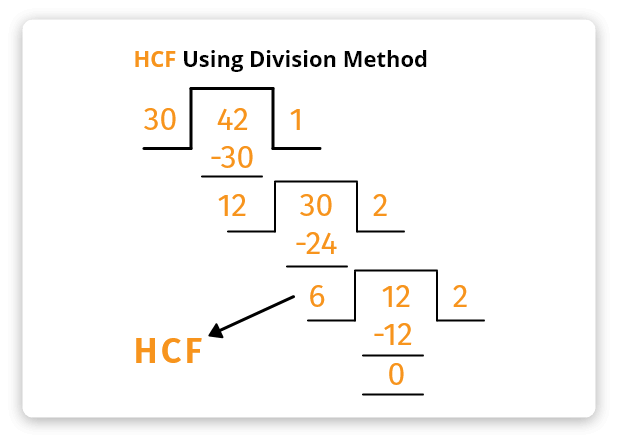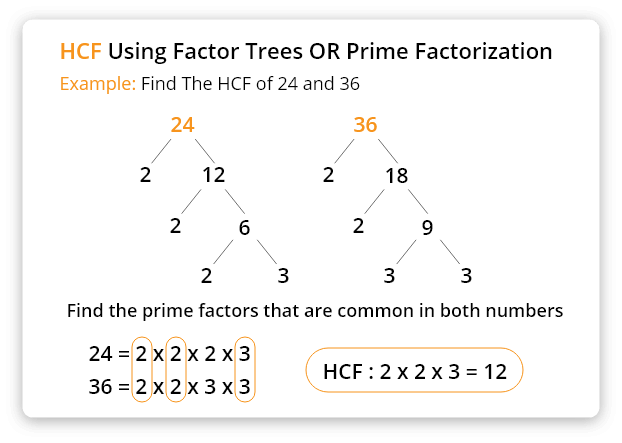# GCF Calculator (HCF Calculator)

Enter up to 10 numbers to calculate Greatest Common Factor (GCF / HCF/ GCD) with steps using our GCF/HCF Calculator.## GCF Calculator

GCF calculator/finder calculates the greatest (highest) common factor of the given numbers using:

Apart from HCF, it also calculates the least common multiple (LCM) for the given numbers.

Note: GCF, GCD, and HCF are the same terms and used to represent the same concept.

In next sections, we will discuss the methods used by the GCD calculator to find the GCF, GCF definition, how to calculate HCF without using the HCF calculator, and few examples to explain how to find the greatest common factor.

## What is GCF?

The Greatest common factor (GCF) of a set of numbers is the biggest factor that all the numbers share. It is commonly known as the highest common factor (HCF).

For example, 8, 12, and 16 have two common factors which are 2 and 4. The greatest is 4. So, the GCF of 8, 12, and 16 is 4.## How to calculate GCF?

If you are already here, you may want to know how to find the GCF. GCF can be calculated by several methods. Below, you can find different ways to calculate the GCF/HCF.

### 1. Factorization method

Example: Find the GCF of 12 and 16 using the factorization method.

Solution:

Factorization or list of factors method uses the factors of given numbers to find the highest common factor.

Step 1: List all factors of the given numbers.

Step 2: Look for the highest common factor.

Refer to the image below for more clarification.### 2. Division Method

Example: Find the GCF of 30 and 42 using the division step method.

Solution:

Step 1: Divide the largest number by the smallest number.

Step 2: Take the divisor from the previous step and divide it with the remainder you got in the previous step.

Step 3: Repeat the 2nd step until the remainder becomes zero. The last divisor will be the highest (greatest) common factor.

Use the HCF finder above to verify the result of your manual calculations. Refer to the below image for the illustration of the division step method.### 3. Prime Factorization

Example: Find the GCF of 24 and 36 using the prime factorization method.

Solution:

Step 1: Make the factors of the given numbers with factor tree, like shown in the image below.

Step 2: Highlight or encircle the common factors of the given numbers.

Step 3: Multiply all common factors to get the GCF. If there is only one common factor, then there is no need to multiply.The greatest common divisor (denominator) calculator enlists all of the steps of the calculation. It is not just a calculation tool. It can also be used to learn the methods to calculate the highest common factor.

## Table of Highest Common Factor.

 GCF of 2 and 4 is 2 GCF of 2 and 5 is 1 GCF of 3 and 4 is 1 GCF of 5 and 25 is 5 GCF of 4 and 5 is 1 GCF of 16 and 24 is 8 GCF of 5 and 7 is 1 GCF of 15 and 20 is 20 GCF of 8 and 12 4 GCF of 8,9 and 25 is 1 HCF of 2 and 3 is 1 HCF of 4 and 8 is 4 GCF of 3,4 and 6 is 1 HCF of 3 and 5 is 1 HCF of 680,510 and 340 is 4 HCF of 2 and 8 is 2 HCF of 18 and 48 is 6 HCF of 12 and 48 is 12 GCF of 30 and 42 is 6